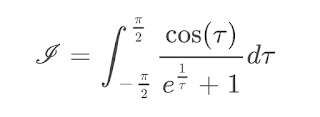### One Symmetric Integral!

Good morning fellow mathematicians! I'm finally back and we are going to dive right in. Today we are going to work with quite the amazing integral, but before we can actually get started, I would like to prove a well-known fact regarding functions. Let us consider some arbitrary function, for example $f:\mathbb{R} \rightarrow \mathbb{R}$. We are now going to go through some simple algebraic manipulations and I hope that you are going to agree with all the steps I'm now doing:

\begin{align*}
f(x)&=\frac{f(x)}{2}+\frac{f(x)}{2}\\
&=\frac{f(x)}{2}+\frac{f(x)}{2}+\frac{f(-x)}{2}-\frac{f(-x)}{2}\\
&=\frac{f(x)+f(-x)}{2}+\frac{f(x)-f(-x)}{2}
\end{align*}

Obviously, adding $f(-x)$ only holds, if we are not leaving the domain of our function. For reasons that will become apparent in a second, we are going to call the first fraction $e(x)$ and the second one $o(x)$, leaving us with the decomposition $f(x)=e(x)+o(x)$. I am now going to claim, that $e(x)$ is an even function, meaning it satisfies $e(-x)=e(x)$, and that $o(x)$ is an odd one, satisfying $o(-x)=-o(x)$. Let us prove the first statement:

\begin{align*}
e(-x)&=\frac{f(-x)+f(-(-x))}{2}\\
&=\frac{f(-x)+f(x)}{2}\\
&=\frac{f(x)+f(-x)}{2}\\
&=e(x)
\end{align*}
$\square$

By the same arguments we can check for the oddity of $o(x)$. This, and the fact that our decomposition is indeed unique, are left as an exercise to the reader to prove.
Now that we have covered the basics, let us move on with the main topic for today:

$$\mathcal{I}=\int_{-\frac{\pi}{2}}^{\frac{\pi}{2}} \frac{\cos (x)}{e^{\frac{1}{x}}+1} d x$$

At the end of the article you are probably going to notice, that today's procedure is going to work for a whole class of special integrals! Now here comes the algebraic part: Let us decompose the integrand into the sum of an odd and even function and use the linearity of the integral to arrive at the big expression:

$$\mathcal{I}=\int_{-\frac{\pi}{2}}^{\frac{\pi}{2}} \frac{1}{2}\left[\frac{\cos (x)}{e^{\frac{1}{x}}+1}+\frac{\cos (-x)}{e^{\frac{-1}{x}}+1}\right] dx +\int_{-\frac{\pi}{2}}^{\frac{\pi}{2}}\frac{1}{2}\left[\frac{\cos (x)}{e^{\frac{1}{x}}+1}-\frac{\cos (-x)}{e^{\frac{-1}{x}}+1}\right]d x$$

This looks way messier than before but trust me, we are going to have a great time now that we have reached this point! Consider for the next part an integral over a symmetric interval, where the integrand is an odd function [just like the second integration part of $\mathcal{I}$] . By introducing the substitution $x\rightsquigarrow-x$ we get:

\begin{align*}
\int_{-a}^{a}f(x)dx&=\int_{a}^{-a}f(-x)(-dx)\\
&=-\int_{-a}^{a}f(x)dx\\

\end{align*}
$$\Longleftrightarrow 2\int_{-a}^{a}f(x)dx=0$$

By trusting the Peano Axioms and the fact that $2\ne0$ we arrive at the obvious conclusion, that an odd function being integrated over a symmetric interval is indeed $0$. This great piece of integration theory also provides us with the second part of $\mathcal{I}$ evaluating to exactly the additive identity, namely:

$$\int_{-\frac{\pi}{2}}^{\frac{\pi}{2}}\frac{1}{2}\left[\frac{\cos (x)}{e^{\frac{1}{x}}+1}-\frac{\cos (-x)}{e^{\frac{-1}{x}}+1}\right]d x = 0$$

In conclusion we've gathered that our original integral is thus basically just its even part being integrated. Continuing from there, I also want you to acknowledge the fact, that $\cos(-x)=\cos(x)$, meaning, we have a common factor which we can drag to the outside:

$$\mathcal{I}= \frac{1}{2}\int_{-\frac{\pi}{2}}^{\frac{\pi}{2}}\cos (x)\left[\frac{1}{e^{\frac{1}{x}}+1}+\frac{1}{e^{\frac{-1}{x}}+1}\right] dx$$

Now by simply expanding the fractions by their denominators respectively, we get:

\begin{align*}
\mathcal{I}&= \frac{1}{2}\int_{-\frac{\pi}{2}}^{\frac{\pi}{2}}\cos (x)\left[\frac{e^{\frac{-1}{x}}+1}{(e^{\frac{1}{x}}+1)(e^{\frac{-1}{x}}+1)}+\frac{e^{\frac{1}{x}}+1}{(e^{\frac{-1}{x}}+1)(e^{\frac{1}{x}}+1)}\right] dx\\
&=\frac{1}{2}\int_{-\frac{\pi}{2}}^{\frac{\pi}{2}}\cos (x)\left[\frac{e^{\frac{-1}{x}}+1+e^{\frac{1}{x}}+1}{(e^{\frac{-1}{x}}+1)(e^{\frac{1}{x}}+1)}\right] dx\\
&=\frac{1}{2}\int_{-\frac{\pi}{2}}^{\frac{\pi}{2}}\cos (x)\left[\frac{e^{\frac{-1}{x}}+1+e^{\frac{1}{x}}+1}{e^{\frac{-1}{x}}e^{\frac{1}{x}}+e^{\frac{1}{x}}+e^{\frac{-1}{x}}+1}\right] dx\\
&=\frac{1}{2}\int_{-\frac{\pi}{2}}^{\frac{\pi}{2}}\cos (x)\left[\frac{e^{\frac{-1}{x}}+1+e^{\frac{1}{x}}+1}{1+e^{\frac{1}{x}}+e^{\frac{-1}{x}}+1}\right] dx\\
&=\frac{1}{2}\int_{-\frac{\pi}{2}}^{\frac{\pi}{2}}\cos (x)dx\\
&=\frac{1}{2}\sin(x)\Biggr|_{-\frac{\pi}{2}}^{\frac{\pi}{2}}\\
&=1
\end{align*}

What a great result! Suddenly the multiplicative identity pops up out of nowhere!

Jokes aside, algebraic manipulation and using symmetry is a crazy powerful tool to evaluate definite integrals. Like mentioned before, we can actually extend this result to a bigger class of integrals and the conditions for those to be evaluated in this very specific way have been given here by this example.

I hope you did enjoy the read and if you did, make sure to subscribe to the blog or activate the push notifications to stay updated! I thank you guys for your attention and up until the next post,

--have a flammable day $\int d \tau$1.2.How to solve millionth order equations ?

3.My favorite parr was the multiplicative identity poping up out of nowhere. What was yours?

4.I think in the case you took, it was enough to just add back I(-x) to I(x), to get the result.